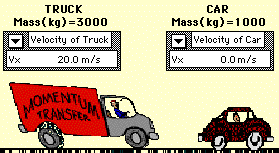## Truck Rear Ends Car

### Inelastic Collision

This animation depicts an inelastic collision between a truck and a car. In the collision between the truck and the car, total system momentum is conserved. Before the collision, the momentum of the truck is 60000 kg*m/s and the momentum of the car is 0 kg*m/s; the total system momentum is 60000 kg*m/s. After the collision, the momentum of the truck is 45000 kg*m/s and the momentum of the car is 15000 kg*m/s; the total system momentum is 60000 kg*m/s. Consistent with the law of momentum conservation, the total system momentum is conserved. The momentum lost by the truck (15000 kg*m/s) is gained by the car.

An analysis of the kinetic energy of the two vehicles reveals that the total system kinetic energy before the collision is 600000 Joules (600000 J for the truck plus 0 J for the car). After the collision, the total system kinetic energy is 450000 Joules (337500 J for the truck and 112500 J for the car). The total kinetic energy before the collision is not equal to the total kinetic energy after the collision. A portion of the kinetic energy is converted to other forms of energy such as sound energy and thermal energy. A collision in which total system kinetic energy is not conserved is known as an inelastic collision.View Mac Version || View Windows Version